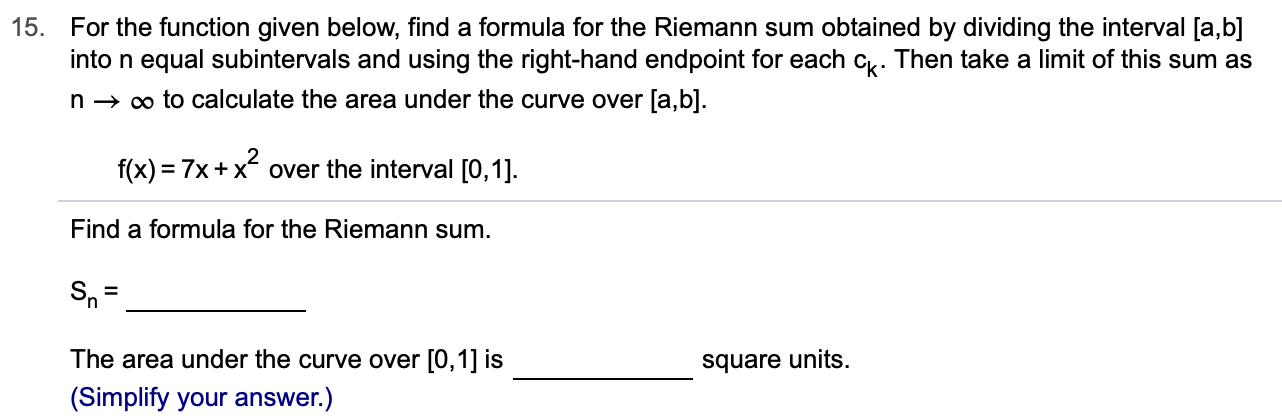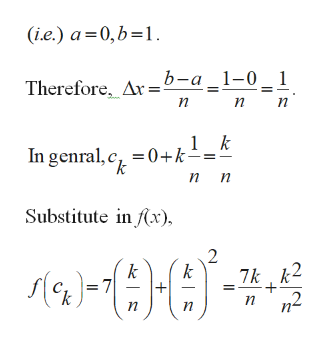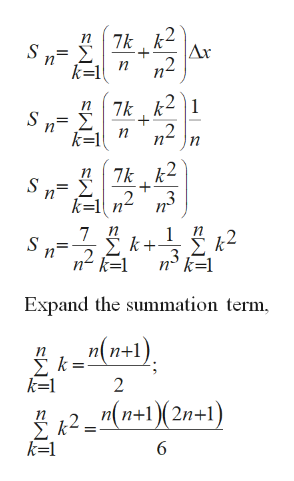# 15.For the function given below, find a formula for the Riemann sum obtained by dividing the interval [a,b]into n equal subintervals and using the right-hand endpoint for each c. Then take a limit of this sum asoo to calculate the area under the curve over [a,b]nf(x) 7x+xover the interval [0,1]Find a formula for the Riemann sumSnThe area under the curve over [0,1] issquare units(Simplify your answer.)

Question
41 views

Can i get help step by step with this problem?help_outlineImage Transcriptionclose15. For the function given below, find a formula for the Riemann sum obtained by dividing the interval [a,b] into n equal subintervals and using the right-hand endpoint for each c. Then take a limit of this sum as oo to calculate the area under the curve over [a,b] n f(x) 7x+xover the interval [0,1] Find a formula for the Riemann sum Sn The area under the curve over [0,1] is square units (Simplify your answer.) fullscreen
check_circle

Step 1

Find the Riemann sum and the area under the curve [0, 1].

Step 2

From the given interval, [0, 1].help_outlineImage Transcriptionclose(i.e.) a 0,b 1 b-а _1-0- 1 Therefore Ar= п п 1 k In genral, c 0+k- п п Substitute in x) 2 7k 2 n2 k 7 k Ле) п п п fullscreen
Step 3

Apply known values in...help_outlineImage Transcriptionclose7k k2 S n 7k k21 n n k=1 7k k2 ,2 3 n S n k-1 n 7 1 k+ k=1 n° k=1 Expand the summation term n(n+1) Σk- k=1 2 2 n+1(2n+1) fullscreen

### Want to see the full answer?

See Solution

#### Want to see this answer and more?

Solutions are written by subject experts who are available 24/7. Questions are typically answered within 1 hour.*

See Solution
*Response times may vary by subject and question.
Tagged in

### Integration